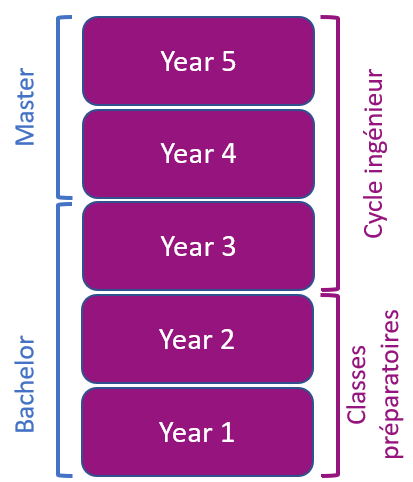# Applied Mathematics - 3AMMA360

A+Augmenter la taille du texteA-Réduire la taille du texteImprimer le documentEnvoyer cette page par mail cet article Facebook Twitter Linked In
• #### Number of hours

• Lectures : 15.0
• Tutorials : 12.0
• Laboratory works : -
• Projects : -
• Internship : -
• Written tests : -
ECTS : 2.5
• Officials : Pierre Alain TOUPANCE

### Goals

The Mathematics for Signal Processing section consists in mastering the mathematical tools that will be used in the AC360 course.
The probability part is an introduction to the study of continuous random models and to the statistical description of populations. In the field of probability, the emphasis will be on formalizing and formulating problems (equations) in probabilistic form, using simple examples from everyday life or elementary technical problems. In statistics, the focus will be on defining the significant characteristics of a population of data (moments, confidence intervals, correlations, etc.) that allow an engineer to understand their meaning, to grasp their limits and to analyze them before making decisions. This course is common to both courses.

Content

Mathematical operators for signal processing + Continuous probability.

• Mathematics for signal processing*
• Continuous probabilities

** Continuous random variables
** Pairs of random variables
** Convergence of sequences of random variables
** Estimation and confidence intervals

Prerequisites

Undergraduate mathematics (integration, series, discrete probabilities)

Tests

E1 : Exam of session 1 : Written 1h30, calculator allowed and the authorized documents are the tables of the normal law, the Student's law and the Chi-square law.
E2 : Exam of session 2 : Written 1h30, calculator allowed and the authorized documents are the tables of the normal law, the Student's law and the Chi-square law.
CC is the grade of the continuous control
In case of confinement, we will keep the same evaluations but at a distance.

Translated with www.DeepL.com/Translator (free version)

Calendar

The course exists in the following branches:

see the course schedule for 2022-2023

Course ID : 3AMMA360
Course language(s):The course is attached to the following structures:

You can find this course among all other courses.

Bibliography

• Bergounioux - Mathématiques pour le traitement du signal - Dunod
• Max et Lacoume - Méthodes et techniques du traitement du signal
• Olivier Rioul - Théorie des probabilités - Hermés

A+Augmenter la taille du texteA-Réduire la taille du texteImprimer le documentEnvoyer cette page par mail cet article Facebook Twitter Linked In

Date of update April 7, 2021

## French engineering curriculum